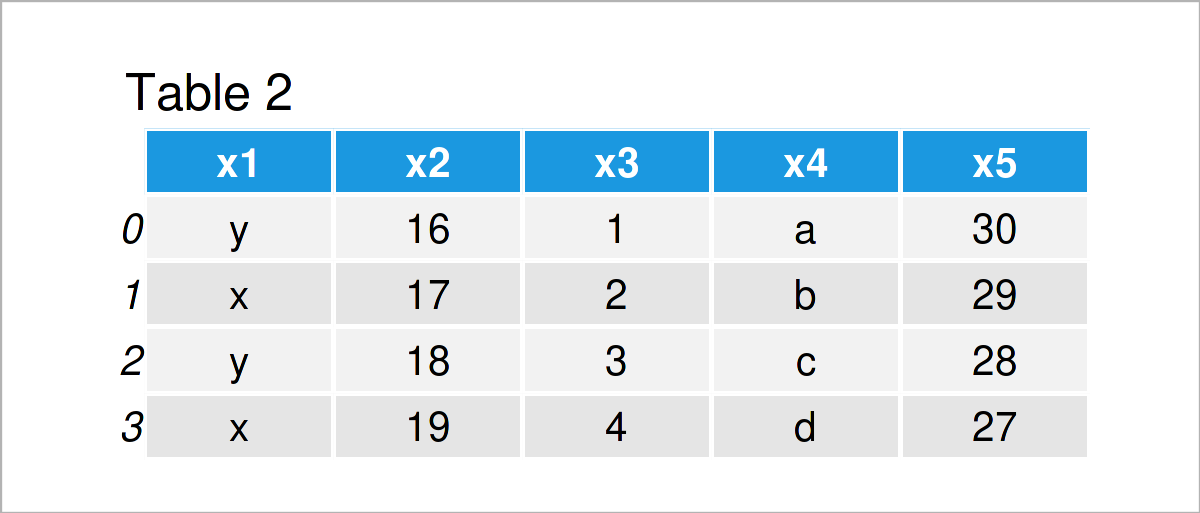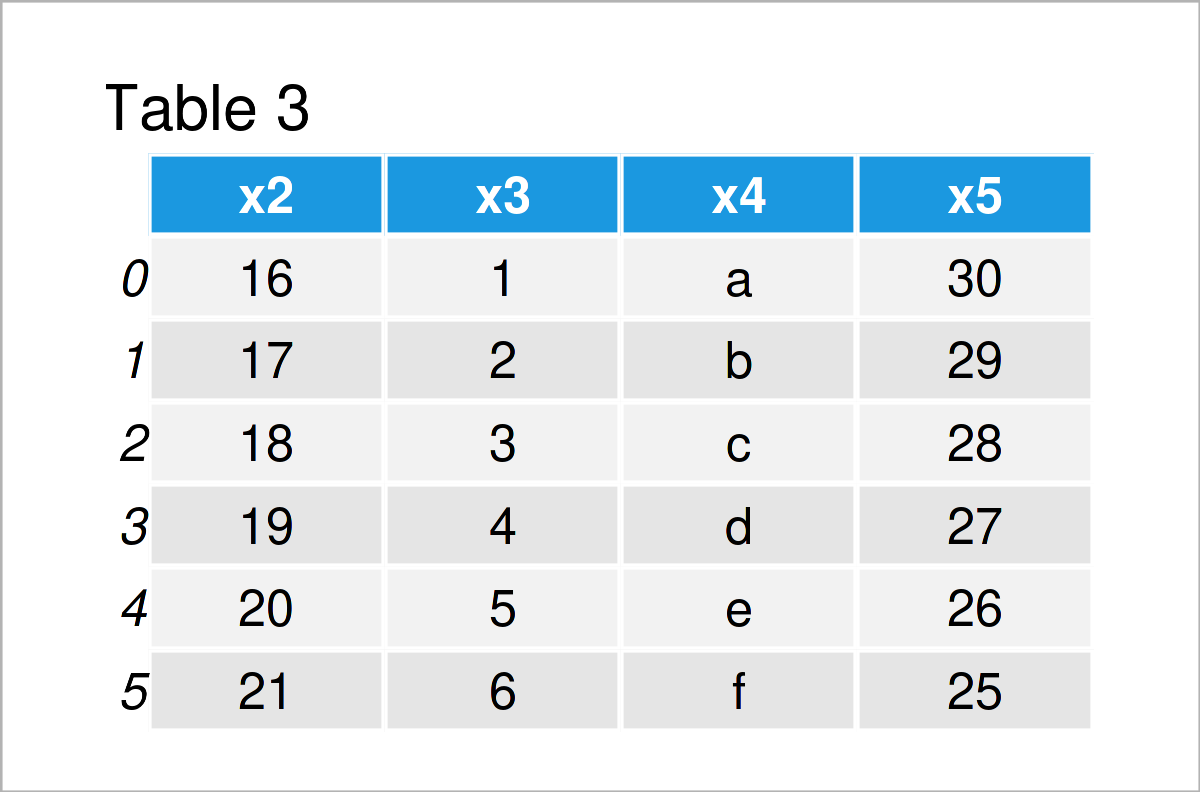# pandas Code in Python (3 Examples)

In this Python tutorial you’ll learn how to apply the functions of the pandas library.

The content looks as follows:

Let’s dive into it.

To be able to use the functions and commands of the pandas library, we first need to import pandas:

`import pandas as pd                                        # Import pandas library to Python`

After executing the previous syntax, we can apply the functions and commands that are provided by the pandas software package.

I’ll show some examples for this now!

## Creating a pandas DataFrame

The pandas library enables the user to create new DataFrames using the DataFrame() function.

Have a look at the following pandas example syntax:

```data = pd.DataFrame({"x1":["y", "x", "y", "x", "x", "y"],  # Construct a pandas DataFrame
"x2":range(16, 22),
"x3":range(1, 7),
"x4":["a", "b", "c", "d", "e", "f"],
"x5":range(30, 24, - 1)})
print(data)                                                # Print pandas DataFrame```As you can see based on Table 1, we have created a DataFrame made of six rows and five columns. Some of these columns are numeric, and some of these columns contain characters.

Next, I’ll show some examples on how to manipulate our pandas DataFrame in Python.

## Example 1: Delete Rows from pandas DataFrame in Python

In Example 1, I’ll illustrate how to remove some of the rows from our data set based on a logical condition.

The Python code below keeps only the rows where the column x2 is smaller than 20:

```data_row = data[data.x2 < 20]                              # Remove particular rows
print(data_row)                                            # Print pandas DataFrame subset```In Table 2 it is shown that we have managed to create a new pandas DataFrame with fewer rows by executing the previous Python code.

## Example 2: Remove Column from pandas DataFrame in Python

Example 2 demonstrates how to drop a column from a pandas DataFrame.

To achieve this, we can use the drop function as shown below:

```data_col = data.drop("x1", axis = 1)                       # Drop certain variable from DataFrame
print(data_col)                                            # Print pandas DataFrame subset```As shown in Table 3, the previous Python programming syntax has created another pandas DataFrame where the column x1 was dropped.

## Example 3: Compute Median of pandas DataFrame Column in Python

It is also possible to perform descriptive analyses based on a pandas DataFrame

This example syntax shows how to calculate the median of the variable x5:

```data_med = data["x5"].median()                             # Calculate median
print(data_med)                                            # Print median
# 27.5```

As you can see, the median value of the variable x5 is 27.5.

## Video & Further Resources

Do you need more information on the content of this tutorial? Then I recommend watching the following video on my YouTube channel. In the video, I explain the topics of this tutorial.

In addition to the video, you might read the related Python articles on this website:

In this Python tutorial you have learned how to use the functions of the pandas library. Let me know in the comments section, if you have further questions or comments. Furthermore, don’t forget to subscribe to my email newsletter in order to receive updates on new articles.

Subscribe to the Statistics Globe Newsletter